Home > ACC7 > Chapter cc310 > Lesson cc310.1.2 > Problem10-28

10-28.
1. Find the length of the side labeled x in each of the right triangles pictured below. Identify each answer as a rational or irrational number. Homework Help ✎

2.  a.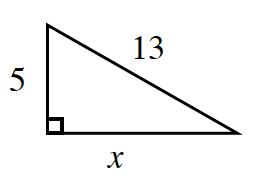b.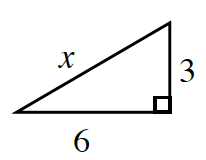c.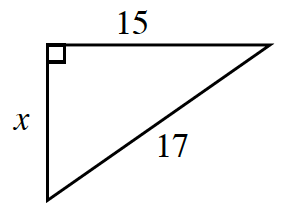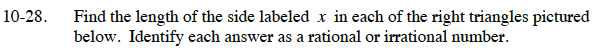Use the Pythagorean Theorem.(leg 1)² + (leg 2)² = (hypotenuse)²
Substitute the values that are given.

(5)² + (x)² = (13)²
25 + x² = 169

Solve by isolating x.

x² = 144
Take the square root of each side to get the answer.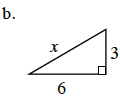(leg 1)² + (leg 2)² = (hypotenuse)²
Substitute the values that are given.

(6)² + (3)² = (x
Now solve for x.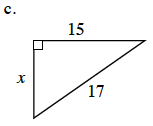See part (a).

8